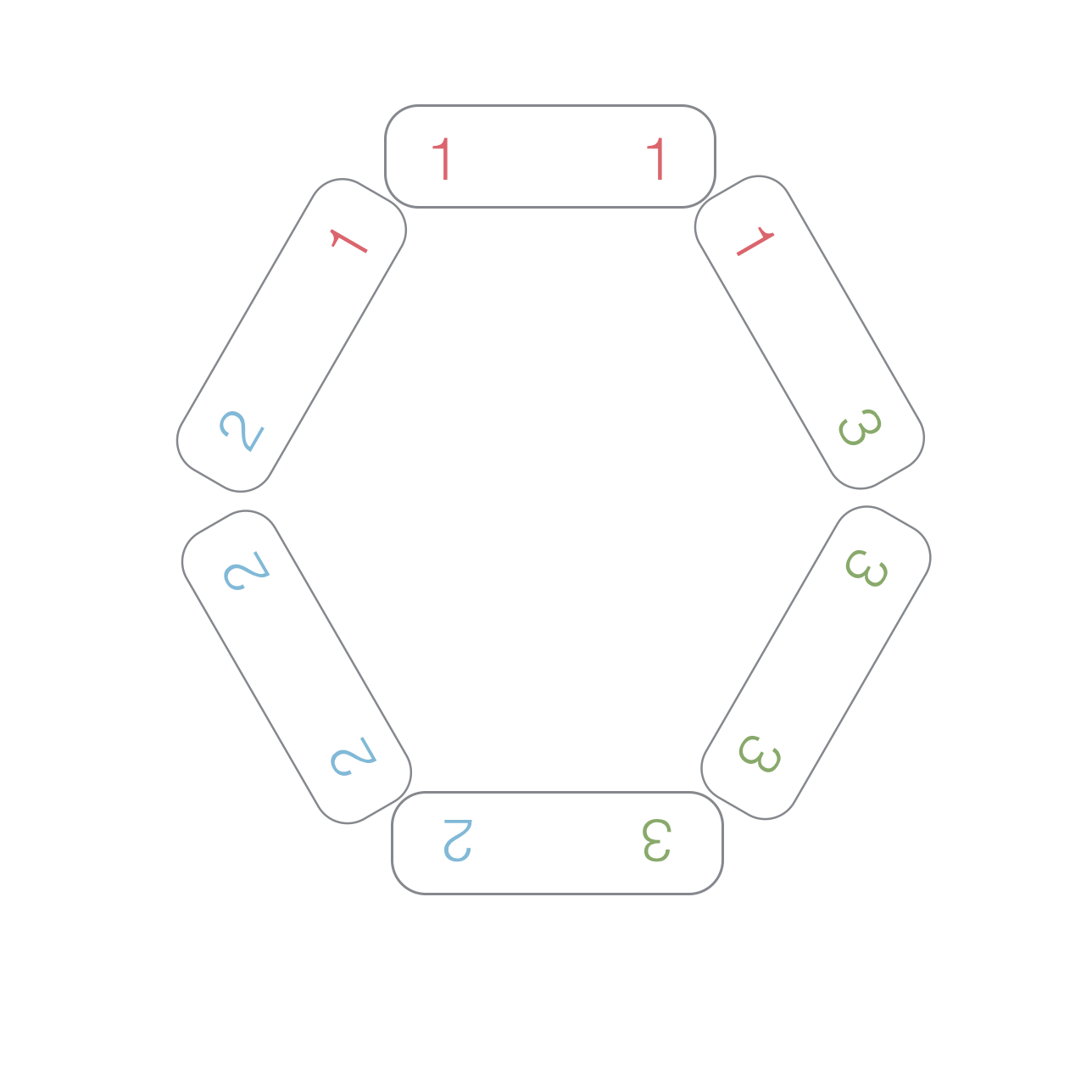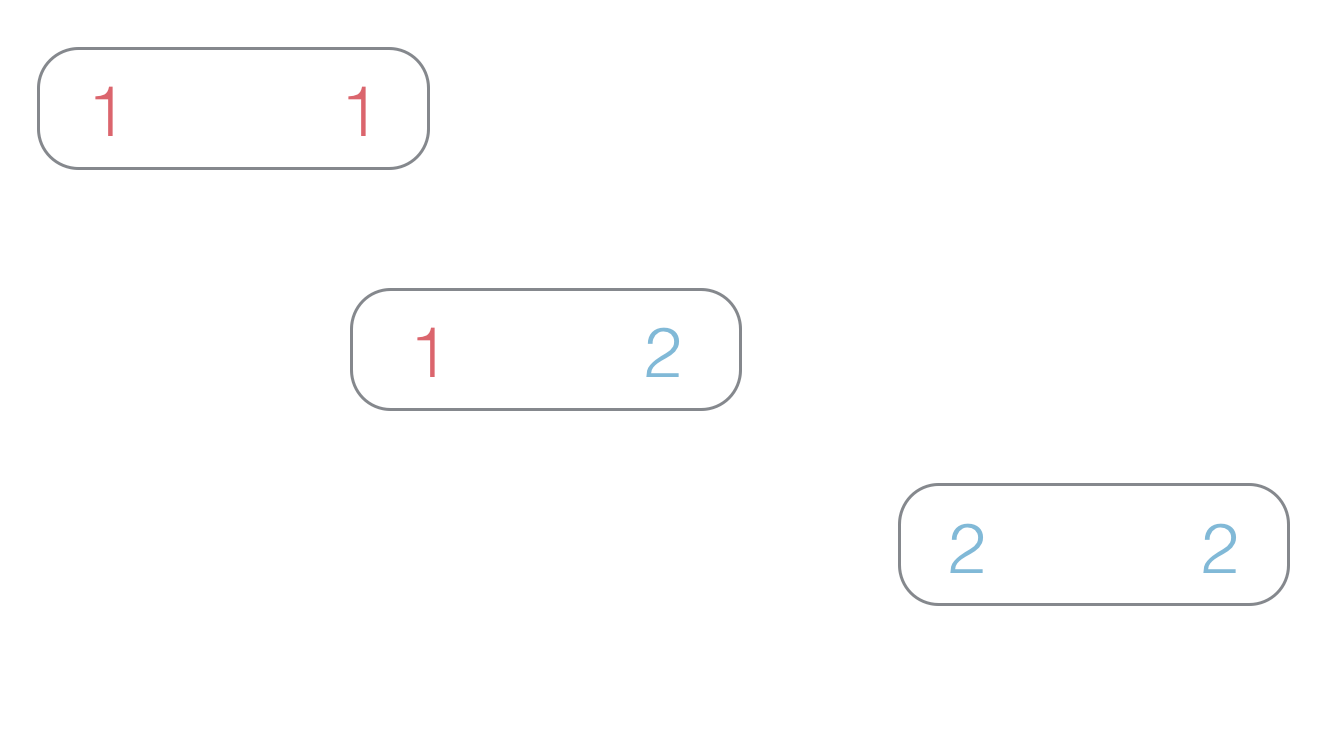# On the Domino Ring

Probability Level 3Consider a set of $\frac{ N (N+1) } { 2 }$ dominos which have faces of values 1 to N.
A ring of dominos is a closed loop of them, in which any 2 touching dominos display the same value.
Let $F(N)$ be the minimum numbers of rings that are needed to use up all of these dominos.

Find the last three digits of $\displaystyle \sum _{n = 1} ^{2016} F(n)$.

Inspiration

• As an explicit example, $F(3) = 1$ because we have the ring listed in the above image.
• For the purposes of this question, assume a single domino to be a ring. So $F(2) = 3$ (see image below) because there are three rings.×